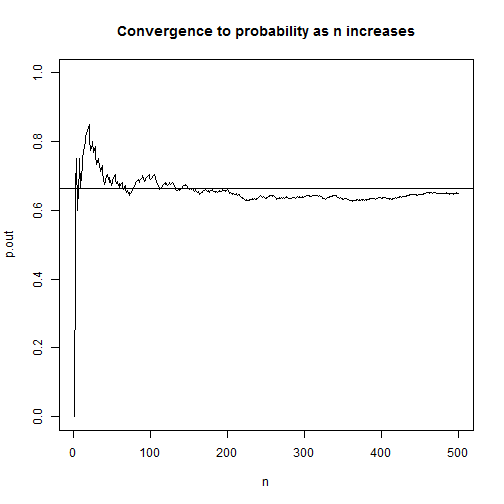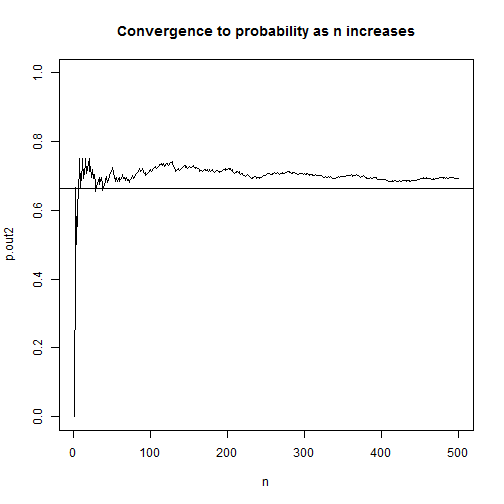# Revisiting some old R-code

One of my first blog posts on this site simulated rolling a die 6 times and observing if side i was observed on the ith^ roll at least once. (For example, rolling a 3 on my 3rd roll would be a success.) I got the idea from one of my statistics textbooks which had a nice picture of the simulation converging to the true probability of 0.665 over the course of 500 trials. I was able to recreate the simulation in R and I guess I got excited and blogged about it. I was reminded of this today when I opened that old textbook and came across the R code that I had actually written in the margin by hand. Apparently I was super proud of my ground-breaking R code and decided to preserve it for posterity in writing. smh

Over 5 years later my precious R code looks pretty juvenile. It's way more complicated than it needs to be and doesn't take advantage of R's vectorized calculations. As Venables and Ripley say in MASS, “Users coming to S from other languages are often slow to take advantage of the power of S to do vectorized calculations…This often leads to unnecessary loops.” Indeed. I'm living proof of that.

I shouldn't be too hard on my myself though. I was not yet familiar with functions like replicate and cumsum. And I was more focused on recreating the plot than writing optimal R code. I went with what I knew. And R, so forgiving and flexible, accommodated my novice enthusiasm.

Here is how I would approach the problem today:

r.out <- replicate(n = 500, any(sample(1:6, size = 6, replace = T) == 1:6))
p.out <- cumsum(r.out)/seq(500)
plot(x = seq(500), y = p.out, type = "l", ylim = c(0,1),
main = "Convergence to probability as n increases", xlab = "n")
abline(h = 0.665)On line 1, we use the sample function to “roll a die 6 times” by sampling the numbers 1 – 6, with replacement, 6 times. Then we compare the 6 results with the vector of numbers 1 – 6 using the == operator and use the any function to check if any are TRUE. Next we replicate that 500 times and store the result in r.out. This is a vector of TRUE/FALSE values which R treats numerically as 1 and 0. This means we can use cumsum to find the cumulative sum of successes. To determine the cumulative proportion of successes, we divide each cumulative sum by the trial number. The result is a vector of porportions that should start converging to 0.665. Finally we plot using base R plot and abline.

This is more efficient than my original attempt 5 years ago and better captures the spirit of the simulation. I'm sure 5 years from now if I stumble upon this post I'll have yet another more elegant way to do it. I'm already looking at it thinking, “I should have generalized this with a function, and used ggplot2 to make the graph. And I shouldn't do seq(500) twice.” In fact I know I could have avoided the replicate function by using the fact that there's a probablity of $$\frac{1}{6}$$ of observing side i on the ith^ roll of a die. So I could have used a single rbinom call to do the simulation, like so:

r.out2 <- cumsum(rbinom(n = 500, size = 6, prob = 1/6) > 0)
p.out2 <- r.out2/seq(500)
plot(x = seq(500), y = p.out2, type = "l", ylim = c(0,1),
main = "Convergence to probability as n increases", xlab = "n")
abline(h = 0.665)In this version instead of simulating 6 literal die rolls, we simulate the number of successes in 6 die rolls. We turn each roll of the die into a binomial event: success or failure. The rbinom function allows us to simulate binomial events where size is the number of trials (or rolls in this case) and prob is the probability of success at each trial. So rbinom(n = 1, size = 6, prob = 1/6) would return a number ranging 0 to 6 indicating the number of success. Think of it as flipping 6 coins, each with probability of getting heads as $$\frac{1}{6}$$, and then counting the number of heads we observed. Setting the n argument to 500 replicates it 500 times. After that it's simply a matter of logically checking which outcomes were greater than 0 and using cumsum on the resulting TRUE/FALSE vector.

This version is way faster. I mean way faster. Compare the time it takes it to do each 1,000,000 times:

system.time({
r.out <- replicate(n = 1e6, any(sample(1:6, size = 6, replace = T) == 1:6))
p.out <- cumsum(r.out)/seq(1e6)
})

##    user  system elapsed
##    5.26    0.00    5.26

system.time({
r.out2 <- cumsum(rbinom(n = 1e6, size = 6, prob = (1/6)) > 0)
p.out2 <- r.out2/seq(1e6)
})

##    user  system elapsed
##    0.06    0.00    0.06


It's not even close. Who was the dummy that wrote that first version with replicate?

But does the new faster version reflect the experimental setting better? Not really. Remember, we're demonstrating probability concepts with die rolls in the first chapter of an intro stats textbook. That's probably not the best time to break out rbinom. And the demo was for 500 trials, not 1,000,000. I had to ramp up the trials to see the speed difference. Maybe the “right” R code in this situation is not the fastest version but rather the one that's easier to understand.

This site uses Akismet to reduce spam. Learn how your comment data is processed.